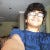## TLDR : Baby Steps

1. prtStrLn : Basically, it prints the given string (and only a string). That’s it.
print : This is like the python print. Converts the given output (of any type) to a string and then prints it. Can supply it anything, and it will show up in the terminal.
2. Basically, functions work differently than in other languages. First, you can supply only one argument. Lovely. I’m not sure how the more complex functions will sort themselves out, but let’s get used to this. Second, declaring functions is different. You have to declare the inputs as follows.
`Code 1add :: Integer -> Integer -> Integeradd x y = x+ymain :: IO ()main = print (add 5 10)`

2. The ‘_’ character is used to denote a value we won’t use (like python).

`Code 2changeTrue :: Bool -> BoolchangeTrue _ = True`

This function basically returns True for every Boolean value we feed in, regardless of initial value.

3. Putting stuff in brackets treats it as a list (Like python).

`Code 3main :: IO ()main = print [changeTrue True , True||False , True&&False , not True]`

The output for the above is True, True, False and False.

4. In Haskell, lists are homogenous. You cannot have a list of different types like integers and bool (unlike python). The following code throws an error in Haskell.

`Code 4main = print [True , 1]`

a. Error : Pattern match(es) are non-exhaustive : This error came when I wrote the function below.

`Code 5changeTrue :: Bool -> BoolchangeTrue False = True`

The reason for this is Bool contains both True and False. Resolved with the following addition.

`Code 6changeTrue :: Bool -> BoolchangeTrue False = TruechangeTrue True = True`

b. Redundant Lambda : The following code gives me redundant lambda and just tells me to use Code 2.

`Code 7changeTrue :: Bool -> BoolchangeTrue = \_ -> True`

c. No instance for (Num Bool) arising from the literal : Basically used if you ever made a heterogenous list of different data types. Homogenise and come back (or something).

--

--I do stuff. Like stuff about policy. And book stuff. And gaming stuff. And stuff about life. And stuff about stuff.